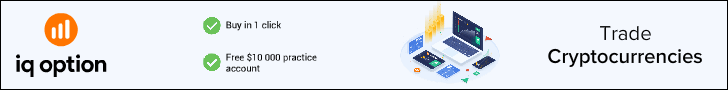# Fibonacci retracement in forexFibonacci retracement levels are used as support and resistance levels. Fibonacci extension levels are used as profit taking levels. Fibonacci retracement in forex, what we will learn today is how to apply Fibonacci tool and how to interpret results that we see on the screen. Fibonacci tool available within their trading platforms.So, click on Fibonacci tool from trading platform that you use. A — our lowest swing, B — our highest swing. So, we will look to BUY some lots at the good lowest price and go up with the trend. Click on A and drag your cursor to B, click. You must see different lines appeared on your chart. Those lines are called Fibonacci Retracement and Extension Levels.

To calculate Fibonacci levels yourself, refer to How to calculate Fibonacci levels. B to some point C, and then continue up in the direction of the trend. Fibonacci retracement levels where we expect the price to take a U-turn and go up again. There we will place our BUY order.

The best situation would be to buy at the lowest level — 0. And on practice the price usually gives us this chance. 500 is also a good level to place a BUY order. Well, let’s take a look at the progress. The price has successfully reached the lowest 0. 618 point and made a U-turn.

So, now when we have our BUY order placed at desired point C, we would like to set some targets to take our profit in the future. 618 extension level, but when the price shows good potential to reach next 1. 618 level, you can leave your trade to get that target too. 618 extension level as our profit target, and according to Figure 2, D is our point for taking profit. Important note: in this Fibonacci tutorial 0. Thus moving further from B would be 1xx. For example: looking at the last picture, if to start counting from point A, then point D would be a 1.

618 Fibonacci extension level or a 161. Forex trading is a high risk investment. All materials are published for educational purposes only. We have created free software — Forex Fibonacci Calculator v2. 1 is a simple and useful tool that will help you to calculate Fibonacci extension and retracement levels for the market price. You will be able to anticipate market price moves and plan future trades according to the calculated results.

Note, that calculation formulas will differ for uptrend and downtrend moves, therefore use appropriate panel in the Calculator to input price values. You can also find those formulas used for calculating Fibonacci levels on the program panel below. In order to calculate Fibonacci levels with this program traders need to fill in High and Low values for the price and click on “Calculate! Tip: Change default field “Decimal places” to get desired number of decimal places for calculated results. Fibonacci calculations can be used for any currency pair and with any time frame. Leonardo Fibonacci is a famous Italian mathematician, founder of a simple series of numbers that refer to ratios valid for natural proportions of things on the planet. If to measure the ratio of any number to one of the next higher number the result will be 0.

618 — are the most important to watch for. Fibonacci Extension Levels are used as targets for taking profit: 0. 618 — here are the most useful for traders. Fibonacci retracement and extension levels carry important information for experienced as well as novice Forex traders as they help to identify entry and exit points during the trade. This chapter will introduce traders to basic rules of applying Fibonacci method in Forex. And now let’s get straight to the point.

Where and how to use Fibonacci study on Forex charts? As you have noticed, there are some A, B, C, D points on the chart. They represent the highest spikes of the price moves or simply highest price swings. To apply Fibonacci study to the price moves we will always look for those points. Forex trading is a high risk investment. All materials are published for educational purposes only. Forex for Beginners Answering all your questions about Forex!